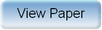Ninth International Geostatistics Congress, Oslo, Norway
June 11 – 15, 2012Session: Plenary 2 Abstract No.: O-003 Title: Non-parametric diagrams for plurigaussian simulations of lithologies Author(s): D. Allard, BioSP, INRA (FR)D. D'Or, Ephesia (CH)P. Biver, TOTAL (FR)R. Froidevaux, Ephesia (CH) Abstract: Although truncated pluri-Gaussian models have been proposed two decades ago, their practical use has been limited by three major impediments: first, the difficulty to relate underlying Gaussian variables to physically interpretable processes; second, the estimation of a truncation diagram from data or concepts is not intuitive for geologists; third, updating a truncation diagram according to current proportions could be a challenge (current practice is to simplify truncation diagrams with rectangular domains). We present an approach for using the truncated bi-Gaussian model when bivariate auxiliary information and lithofacies data are available at wells allowing for very general shapes. The general idea is to relate the Gaussian variables to auxiliary variables and to build truncation diagrams by calibration with lithofacies observed at wells. This general idea is mathematically translated as following: on the basis of every pair A of auxiliary values (e.g. p waves acoustic impedance and Poisson ratio), the conditional probability f(k|A) for each of the k=1,?,K lithofacies is estimated with kernel regression using well data. A truncation diagram is then defined by finding the lithofacies k such that akf(k|A) is maximum, where (a1,?aK) is a vector such that the resulting truncation diagram leads to frequencies of lithofacies equal to the target proportions (1,?,K). This approach enables us to use the truncated bi-Gaussian model in a context of non-stationary target proportions. The paper first details the different steps involved in this approach: kernel regression to estimate conditional probability functions and optimization procedure to obtain the target proportions. It then illustrates the method on a real data set in a mixed carbonate platform environment.Produced by X-CD Technologies Inc.